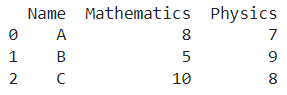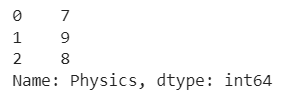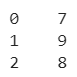# Pretty-print An Entire Pandas Series / DataFrame

A DataFrame is the primary data structure of the Pandas library in Python and is commonly used for storing and working with tabular data.

A common operation that could be performed on such data is to pretty-print an entire Pandas Series / DataFrame to visualize the information in a better manner.

To start working with Pandas, we first need to import it:

``import pandas as pd``

## Running Example

Let us understand this operation with the help of an example. Consider the following DataFrame containing 3 students with names A, B, and C and their corresponding marks (out of 10) for two subjects, Mathematics and Physics.Code snippet for generating the above DataFrame :

### Python 3 Code :

``````import pandas as pd

# Dictionary for our data
data = {'Name' : ['A', 'B', 'C'], 'Mathematics' : [8, 5, 10], 'Physics' : [7, 9, 8]}

# DataFrame for the dictionary
df = pd.DataFrame(data)

# Printing the DataFrame
print(df)``````

Here, data is a dictionary we created to initialize the DataFrame. For this, we use the DataFrame() function of the Pandas library which takes the dictionary as an argument and returns the required DataFrame.

Now, let’s say we need to pretty-print an entire Pandas Series / DataFrame. The resulting output would look like this :Let us look at different ways of performing this operation on a given DataFrame :

## Pretty Print Dataframe/Series by using the Pandas.option_context() function

In this method, we use the Pandas.option_context() function to pretty-print the DataFrame.

This helps us in setting up various display options for printing the DataFrame, which is very useful when it comes to handling DataFrames that are very large in size.

Here, we pass parameters in the form of display options followed by desired values.

In the example below, we passed None for the display options of max_rows and max_columns which essentially turns these upper limit constraints off.

Thus there is no limit to the number of columns and rows that can be displayed. Let us take a look at the corresponding code snippet and generated output for this method:

``````# Importing required libraries
import pandas as pd

# Dictionary for our data
data = {'Name' : ['A', 'B', 'C'], 'Mathematics' : [8, 5, 10], 'Physics' : [7, 9, 8]}

# DataFrame for the dictionary
df = pd.DataFrame(data=data)

# Pretty printing
with pd.option_context('display.max_rows', None, 'display.max_columns', None):
print(df)``````

Output :In a similar manner, we can use this method to print a series of interests, say the physics column of our DataFrame in a pretty manner. We just replace df by df[‘Physics’] to access the physics column. Let us take a look at the corresponding code snippet and generated output for this method :

### Python 3 Code :

``````# Importing required libraries
import pandas as pd

# Dictionary for our data
data = {'Name' : ['A', 'B', 'C'], 'Mathematics' : [8, 5, 10], 'Physics' : [7, 9, 8]}

# DataFrame for the dictionary
df = pd.DataFrame(data=data)

# Pretty printing
with pd.option_context('display.max_rows', None, 'display.max_columns', None):
print(df['Physics'])``````

Output :Instead of using df[‘Physics’] to access the physics column, we could also simply use the dot operator in the form of df.Physics to get the same result.

Let us take a look at the corresponding code snippet and generated output for this method:

``````# Importing required libraries
import pandas as pd

# Dictionary for our data
data = {'Name' : ['A', 'B', 'C'], 'Mathematics' : [8, 5, 10], 'Physics' : [7, 9, 8]}

# DataFrame for the dictionary
df = pd.DataFrame(data=data)

# Pretty printing
with pd.option_context('display.max_rows', None, 'display.max_columns', None):
print(df.Physics)``````

Output:## Pretty Print Dataframe/Series by using the DataFrame.to_string() function

In this method, we use the DataFrame.to_string() function to pretty print a given DataFrame.

This function requires no parameters and returns a structured string object that can be used to print the information stored in the DataFrame in a visually appealing manner.

Let us take a look at the corresponding code snippet and generated output for this method :

``````# Importing required libraries
import pandas as pd

# Dictionary for our data
data = {'Name' : ['A', 'B', 'C'], 'Mathematics' : [8, 5, 10], 'Physics' : [7, 9, 8]}

# DataFrame for the dictionary
df = pd.DataFrame(data=data)

# Pretty printing
print(df.to_string())``````

Output :In a similar manner, we can use this method to print a series of interest, say the physics column of our DataFrame in a pretty manner.

We just replace df by df[‘Physics’] to access the physics column. Let us take a look at the corresponding code snippet and generated output for this method :

``````# Importing required libraries
import pandas as pd

# Dictionary for our data
data = {'Name' : ['A', 'B', 'C'], 'Mathematics' : [8, 5, 10], 'Physics' : [7, 9, 8]}

# DataFrame for the dictionary
df = pd.DataFrame(data=data)

# Pretty printing
print(df['Physics'].to_string())``````

Output:Instead of using df[‘Physics’] to access the physics column, we could also simply use the dot operator in the form of df.Physics to get the same result.

Let us take a look at the corresponding code snippet and generated output for this method :

``````# Importing required libraries
import pandas as pd

# Dictionary for our data
data = {'Name' : ['A', 'B', 'C'], 'Mathematics' : [8, 5, 10], 'Physics' : [7, 9, 8]}

# DataFrame for the dictionary
df = pd.DataFrame(data=data)

# Pretty printing
print(df.Physics.to_string())``````

Output:## Conclusion

In this topic, we have learned to pretty-print an existing Pandas DataFrame, following a running example of test scores of students in different subjects, thus giving us an intuition of how this concept could be applied in real-world situations. Feel free to reach out to [email protected] in case of any suggestions.

Trending